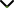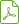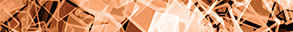# Stability and Bifurcations Analysis of Discrete Dynamical Systems

Publishing date
01 Feb 2019
Status
Published
14 Sep 2018

Guest Editors

2Mansoura University, Mansoura, Egypt

# Stability and Bifurcations Analysis of Discrete Dynamical Systems

## DescriptionThe importance of difference equations cannot be overemphasized. These equations model discrete physical phenomena on one hand and are integral part of numerical schemes used to solve differential equations, on the other hand. This widens the applicability of such equations to many branches of scientific knowledge.

Discrete dynamical systems are described by difference equations and potentially have applications in probability theory, economics, biology, computer science, control engineering, genetics, signal processing, population dynamics, health sciences, ecology, physiology, and physics.

The study of such systems has direct impact on human sciences such as ecosystem, health, population dynamics, and decision-making. This motivated a number of researchers to study the behavior of these systems in recent years. The behavior of system of difference equations means studying equilibrium points, local and global asymptotic stability, semi-conjugacy analysis, prime-period two solutions, rate of convergence, forbidden set, boundedness and persistence, existence and uniqueness of positive equilibrium point, and many more.

The results about these equations pave path towards the development of basic theory of higher-order systems of nonlinear difference equations. If the behavior of a solution, depending on a parameter, changes with the variation in the values of parameter, then a bifurcation occurs. The bifurcation analysis gives more insight about the system under study, especially about the equilibrium points that are sensitive with change of values of parameters.

The aims of this special issue include providing a platform to disseminate original research in the fields of system of difference equations and discrete dynamical systems. This is an excellent opportunity for researchers to share their findings with the scientific community, in general and particularly working in the fields related to the title of the special issue. The researchers can contribute their original work that addresses any aspect of stability and bifurcations analysis of discrete dynamical systems. This special issue includes the study of bifurcation analysis of discrete-time models from economics, biology, ecology, physiology, and physics.

Potential topics include but are not limited to the following:

• Boundedness and persistence of difference equations and systems of difference equations
• Existence and uniqueness of positive equilibrium point
• Local and global asymptotic stability
• Rate of convergence
• Periodicity nature of difference equations and systems of difference equations
• Lyapunov stability analysis
• Bifurcations analysis like Neimark-Sacker or Hopf bifurcation, subcritical and supercritical Neimark-Sacker bifurcation, transcritical bifurcation, period-doubling, and fold bifurcation of some discrete-time models from biology and ecology.Download Special IssueJournal metrics
Acceptance rate26%
Submission to final decision52 days
Acceptance to publication33 days
CiteScore1.800
Journal Citation Indicator0.290
Impact Factor1.348Author guidelinesEditorial boardDatabases and indexing

Article of the Year Award: Outstanding research contributions of 2020, as selected by our Chief Editors. Read the winning articles.